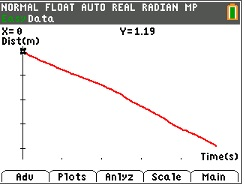### Which Way?

Students' use a motion detector to examine how different types of motion affect the shape of the Distance versus Time plot. They explore how changes in direction and other factors affect the shape of the plot.
•TI-84 Plus CE
•TI-84 Plus C Silver Edition
• TI-84 Plus Silver Edition
• TI-84 Plus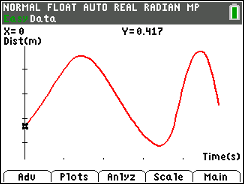### Shape Up!

In this activity, students will use the motion detector to record motion, and observe how the direction of movement, speed of travel, and the rate of change of direction and speed affect the shape of a Distance-Time plot.
•TI-84 Plus CE
•TI-84 Plus C Silver Edition
• TI-84 Plus Silver Edition
• TI-84 Plus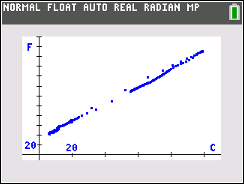### Coming to know F and C

Students will collect room temperatures with temperature probes and graphing calculators, and plot data to generalize rule that relates Fahrenheit and Celsius units.
•TI-84 Plus CE
•TI-84 Plus C Silver Edition
• TI-84 Plus Silver Edition
• TI-84 Plus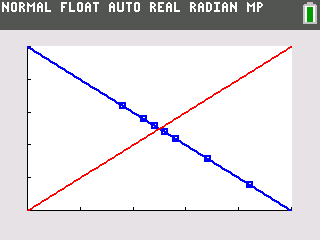### The 1 Cent Event

In this activity, students will collect data on a 1 cent coin as it falls under its own volition after being placed on its edge 25 times. They will report their data to the class and enter the data into their TI-84 Plus. They will examine the data from the collective and discuss patterns revealed in the data.
•TI-84 Plus CE
•TI-84 Plus C Silver Edition
• TI-84 Plus Silver Edition
• TI-84 Plus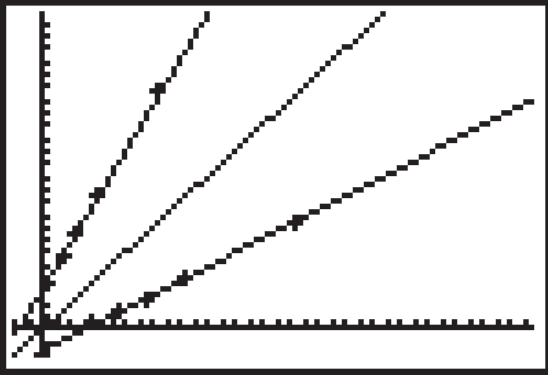### Inverse of Two Temps

Students find a conversion equation that will calculate the corresponding Celsius temperature for any given Fahrenheit temperature. Students learn to graph scatter plots, analyze and graph linear equations, compute and model slope, derive and apply a conversion equation, and analyze inverse relations.
•TI-84 Plus CE
•TI-84 Plus C Silver Edition
• TI-84 Plus Silver Edition
• TI-84 Plus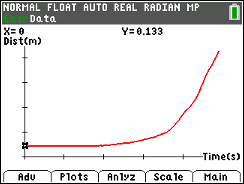### Slow Down - Speed Up

In this activity, students' will use a motion detector to observe the effect of speeding up, slowing down, and moving at a constant rate on a Distance versus Time plot.
•TI-84 Plus CE
•TI-84 Plus C Silver Edition
• TI-84 Plus Silver Edition
• TI-84 Plus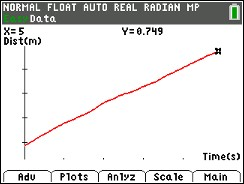### Walk the Line

In this activity, students' will create constant-speed motion plots and develop linear equations to describe them mathematically.
•TI-84 Plus CE
•TI-84 Plus C Silver Edition
• TI-84 Plus Silver Edition
• TI-84 Plus### Swing Thing

In this activity, students will construct a pendulum and create a motion plot for a swinging pendulum. They will also identify characteristics that affect a pendulum's motion.
•TI-84 Plus CE
•TI-84 Plus C Silver Edition
• TI-84 Plus Silver Edition
• TI-84 PlusIn this activity, students' will use a motion detector to determine what effect the speed at which a person moves has on the appearance of the Distance versus Time plot.
•TI-84 Plus CE
•TI-84 Plus C Silver Edition
• TI-84 Plus Silver Edition
• TI-84 Plus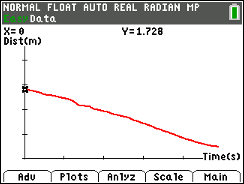### Slippery Slope

In this activity, students will create Distance versus Time plots and calculate the slopes of the plots. They explore the mathematical concept of slopes and understand how slopes can be used to interpret how one physical quantity changes with respect to another.
•TI-84 Plus CE
•TI-84 Plus C Silver Edition
• TI-84 Plus Silver Edition
• TI-84 Plus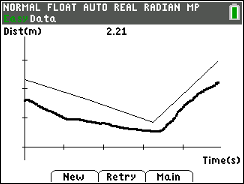### Match Me!

In this activity, students move in a specific way in front of the motion detector to create motion plots that match a given Distance versus Time plot. They make connections between types of movements and characteristics of Distance-Time graphs. They learn to change the shape of the plot by adjusting the starting point, speed, and the direction of motion.
•TI-84 Plus CE
•TI-84 Plus C Silver Edition
• TI-84 Plus Silver Edition
• TI-84 Plus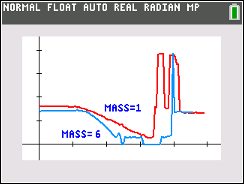### Falling Down

In this activity, students' will determine the average speed of a falling object. They will observe whether or not changing the mass and keeping the same shape have an effect on the average speed of the object.
•TI-84 Plus CE
•TI-84 Plus C Silver Edition
• TI-84 Plus Silver Edition
• TI-84 Plus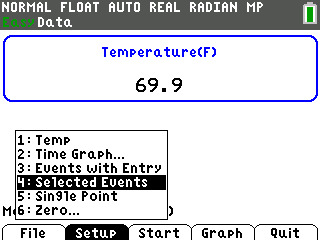### Two Hot, Two Cold

In this activity, students collect both Celsius and Fahrenheit temperature data for several water samples of varying temperatures. They use the data to find a conversion equation that will calculate the Fahrenheit temperature for any given Celsius temperature.
•TI-84 Plus CE
•TI-84 Plus C Silver Edition
• TI-84 Plus Silver Edition
• TI-84 Plus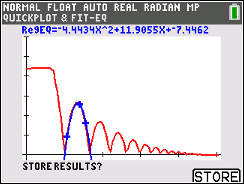### Curve Ball

In this activity, students' will create a Height-Time plot for a bouncing ball and use a quadratic equation to describe the ball's motion.
•TI-84 Plus CE
•TI-84 Plus C Silver Edition
• TI-84 Plus Silver Edition
• TI-84 Plus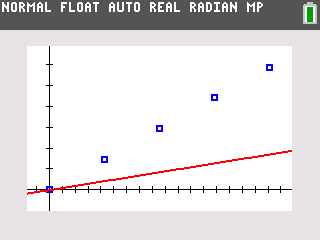### Making Cents of Density

In this activity, students will measure the mass and volume of several collections of a coin type and enter the data into their handhelds and explore for patterns. Students will also make a plot of mass vs. volume and create a mathematical model that demonstrates the relationship between mass and volume.
•TI-84 Plus CE
•TI-84 Plus C Silver Edition
• TI-84 Plus Silver Edition
• TI-84 Plus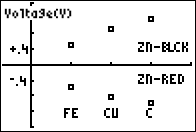### Vernier - Lemon "Juice"

"Juice" is a slang term sometimes used for electricity. Batteries are made up of one or more cells. Cells often consist of two different materials in a solution that are connected to each other by a wire. In this experiment, you will study some basic principles of cells using the juice of a lemon as the cell solution. You will place small pieces of two different materials into the lemon, and a calculator will be used to measure and display the voltages produced.
•TI-84 Plus CE
•TI-84 Plus C Silver Edition
• TI-84 Plus Silver Edition
• TI-84 Plus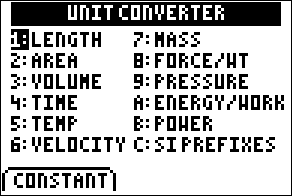### An ounce of prevention

Students will collect measures off of containers for the volume in both milliliters and ounces. They will set up a plot to determine the relationship between the two measures. Students will predict values for volumes in one unit, given the other.
•TI-84 Plus CE
•TI-84 Plus C Silver Edition
• TI-84 Plus Silver Edition
• TI-84 Plus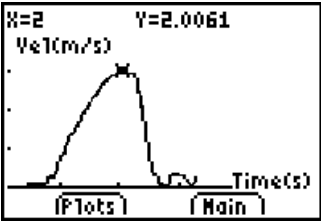### Vernier - A Speedy Slide with EasyData™ App and CBR 2™

Students will use a CBR 2™ motion detector to determine their speed or velocity going down a playground slide. They will also experiment with different ways to increase their speed going down the slide.
•TI-84 Plus CE
•TI-84 Plus C Silver Edition
• TI-84 Plus Silver Edition
• TI-84 Plus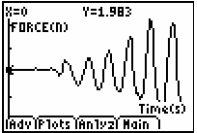### Vernier - Friction

Students study how the nature of two materials in contact, and the smoothness of their surfaces affect the magnitude of the sliding force of friction. They use a force sensor to measure frictional force for different surfaces.
•TI-84 Plus CE
•TI-84 Plus C Silver Edition
• TI-84 Plus Silver Edition
• TI-84 Plus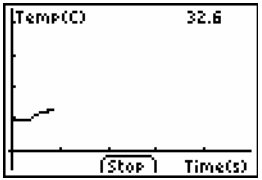### Vernier - Heat of Fusion

In this activity, students will use a calorimeter to measure the amount of heat needed to melt ice and determine the heat of fusion for ice. EasyData™ is needed for this activity.
•TI-84 Plus CE
•TI-84 Plus C Silver Edition
• TI-84 Plus Silver Edition
• TI-84 Plus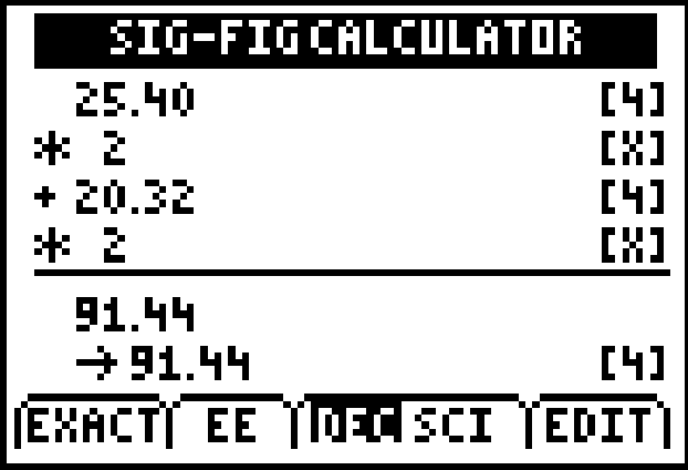### How Do Your Errors Grow!

A simple measuring activity allows students to explore the sometimes difficult concept of significant figures, both manually and with the help of the Science Tools App's Sig-Fig Calculator.
•TI-84 Plus CE
•TI-84 Plus C Silver Edition
• TI-84 Plus Silver Edition
• TI-84 Plus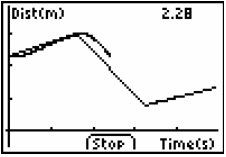••